# Half Subtractor Circuit Construction using Logic Gates

Half subtractor is the most essential combinational logic circuit which is used in digital electronics. Basically, this is an electronic device or in other terms, we can say it as a logic circuit. Half subtractor is used to perform two binary digits subtraction. In the previous article, we have already discussed the concepts of half adder and a full adder circuit which uses the binary numbers for the calculation. Similarly, the subtractor circuit uses binary numbers (0,1) for the subtraction. The circuit of the half subtractor can be built with two logic gates namely NAND and EX-OR gates. This circuit gives two elements such as the difference as well as the borrow. This article gives half subtractor theory concept which includes theories like what is a subtractor, half subtractor with the truth table, etc.

## What is a Half Subtractor?

Before going to discuss the half subtractor, we have to know the binary subtraction. In binary subtraction, the process of subtraction is similar to arithmetic subtraction. In arithmetic subtraction the base 2 number system is used whereas in binary subtraction, binary numbers are used for subtraction. The resultant terms can be denoted with the difference and borrow.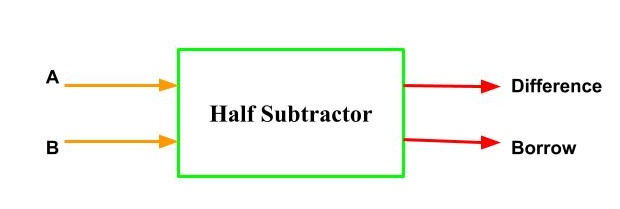Half Subtractor Block Diagram

As in binary subtraction, the major digit is 1, we can generate borrow while the subtrahend 1 is superior to minuend 0 and due to this, borrow will need. The following example gives the binary subtraction of two binary bits.

 First Digit Second Digit Difference Borrow 0 0 0 0 1 0 1 0 0 1 1 1 1 1 0 0

In the above subtraction, the two digits can be represented with A and B. These two digits can be subtracted and gives the resultant bits as difference and borrow.

When we observe the first two and fourth rows, the difference of these rows, then the difference and borrow are similar because subtrahend is lesser than the minuend. Similarly, when we observe the third row, the minuend value is subtracted from the subtrahend. So the difference and borrow bits are 1 because the subtrahend digit is superior to the minuend digit.

## Half-Subtractor Block Diagram

The block diagram of the half subtractor is shown above. It requires two inputs as well as gives two outputs. Here inputs are represented with A&B, and outputs are Difference and Borrow.

The above circuit can be designed with EX-OR & NAND gates. Here, NAND gate can be build by using AND and NOT gates. So we require three logic gates for making half subtractor circuit namely EX-OR gate, NOT gate, and NAND gate.

Combination of AND and NOT gate produce a different combined gate named as NAND Gate. The Ex-OR gate output will be the Diff bit and the NAND Gate output will be the Borrow bit for the same inputs A&B.

### AND-Gate

The AND-gate is one type of digital logic gate with multiple inputs and a single output and based on the inputs combinations it will perform the logical conjunction. When all the inputs of this gate are high, then the output will be high otherwise the output will be low. The logic diagram of AND gate with truth table is shown below.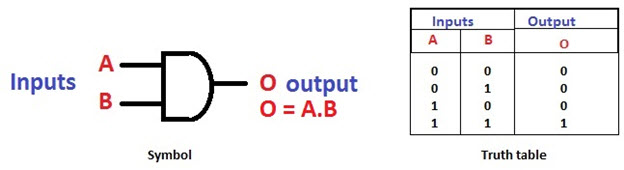AND Gate and its Truth Table

### NOT Gate

The NOT-gate is one type of digital logic gate with a single input and based on the input the output will be reversed. For instance, when the input of the NOT gate is high then the output will be low. The logic diagram of NOT-gate with truth table is shown below. By using this type of logic gate, we can execute NAND and NOR gates.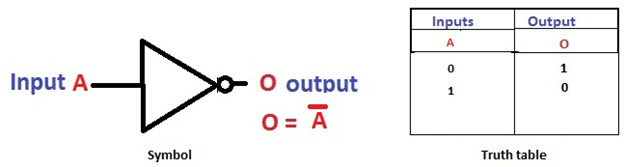NOT Gate and its Truth Table

### Ex-OR Gate

The Exclusive-OR or EX-OR gate is one type of digital logic gate with 2-inputs & single output. The working of this logic gate depends on OR gate. If any one of the inputs of this gate is high, then the output of the EX-OR gate will be high. The symbol and truth table of the EX-OR are shown below.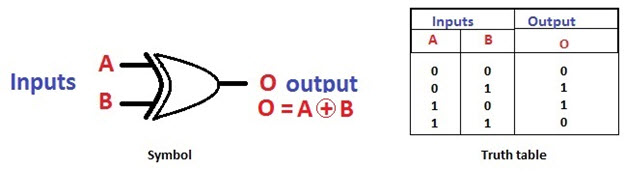EXOR Gate and its Truth Table

## Half Subtractor Circuit using Nand Gate

The designing of half subtractor can be done by using logic gates like NAND gate & Ex-OR gate. In order to design this half subtractor circuit, we have to know the two concepts namely difference and borrow.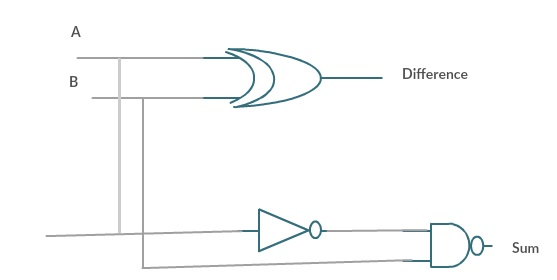Half Subtractor Circuit using Nand Gate

If we monitor cautiously, it is fairly clear that the variety of operation executed by this circuit which is accurately related to the EX-OR gate operation. Therefore, we can simply use the EX-OR gate for making difference. In the same way, the borrow produced by half adder circuit can be simply attained by using the blend of logic gates like AND- gate and NOT-gate.

### Truth Table

The truth table of the half adder circuit is shown below. It is an essential tool for any kind of digital circuit to know the possible combinations of inputs and outputs. For instance, if the subtractor has two inputs then the resultant outputs will be four. The o/p of the half subtractor is mentioned in the below table that will signify the difference bit as well as borrow bit. The half subtractor truth table explanation can be done by using the logic gates like EX-OR logic gate and AND gate operation followed by NOT gate.

 First Bit Second Bit Difference (EX-OR Out) Borrow (NAND Out) 0 0 0 0 1 0 1 0 0 1 1 1 1 1 0 0

Solving the truth table using K-Map is shown below.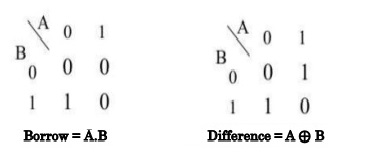half subtractor k map

The Boolean expression of the half subtractor using truth table and K-map can be derived as

Difference (D) = (x’y + xy’)

= x ⊕ y
Borrow (B) = x’y

#### Application of Half Subtractor

The applications of half subtractor include the following.

• Half subtractor is used to reduce the force of audio or radio signals
• It can be used in amplifiers to reduce the sound distortion
• Half subtractor is used in ALU of processor
• It can be used to increase and decrease operators and also calculates the addresses
• Half subtractor is used to subtract the least significant column numbers. For subtraction of multi-digit numbers, it can be used for the LSB.

Therefore, from the above half subtractor theory, at last, we can close that by using this circuit we can subtract from one binary bit from another to provide the outputs like Difference and Borrow. Similarly, we can design half subtractor using NAND gates circuit as well as NOR gates. Here is a question for you, what are universal logic gates?

Photo Credits

Half Subtractor blogspot